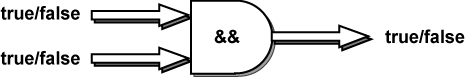```How much flour do you have? 6
How much sugar do you have? 4
```

When execution gets to the `if` statement, it finds that

```flour >= 4
```

is true, because 6 >= 4. And

```sugar >= 2
```

is true, because 4 >= 2 Both sides are true, so AND gives true.

# AND OperatorThe and operator requires that both sides are true:

this side must be true && this side must be true

If both sides are true, the entire expression is true. If either side (or both) are false, the entire expression is false. && is a logical operator because it combines two true/false values into a single true/false value.

Here is what && does:

• `true  && true  = true`
• `false && true  = false`
• `true  && false = false`
• `false && false = false`

Use and when every requirement must be met.

### QUESTION 5:

Look at the boolean expression:

```flour >= 4 && sugar >= 2
```

What will the expression give us if `flour` is 2 and `sugar` is 0?